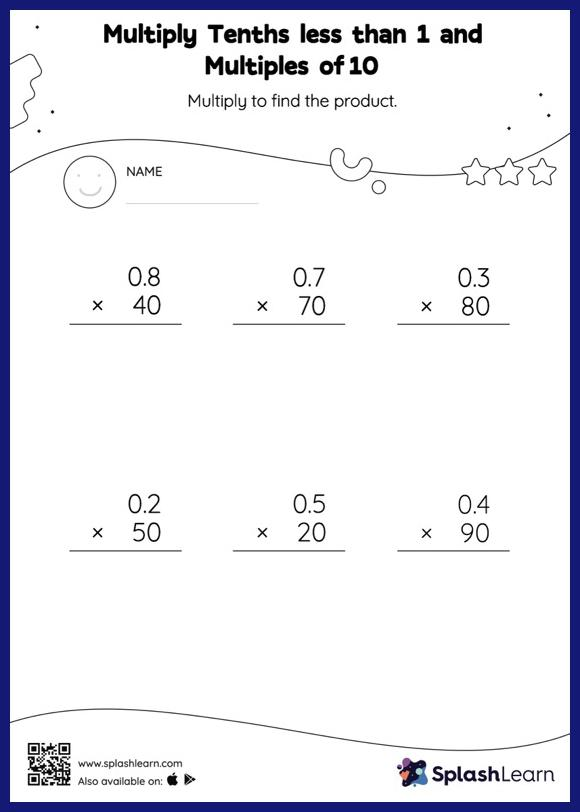# Multiply Tenths less than 1 and Multiples of 10: Vertical Multiplication Worksheet

Home > Multiply Tenths less than 1 and Multiples of 10: Vertical MultiplicationStudents demonstrate their problem-solving ability as they work on this multiply tenths less than 1 and multiples of 10 worksheet. When students multiply a decimal by a number ending in zeros, they first ignore the decimal point, multiply the numbers, and then add the decimal point in the product as many places from the right as the number of decimal digits in the original number. In this worksheet, students practice solving problems using the column method. This method is especially helpful with problems involving bigger multi-digit numbers as the format provides an easy structure to follow.